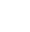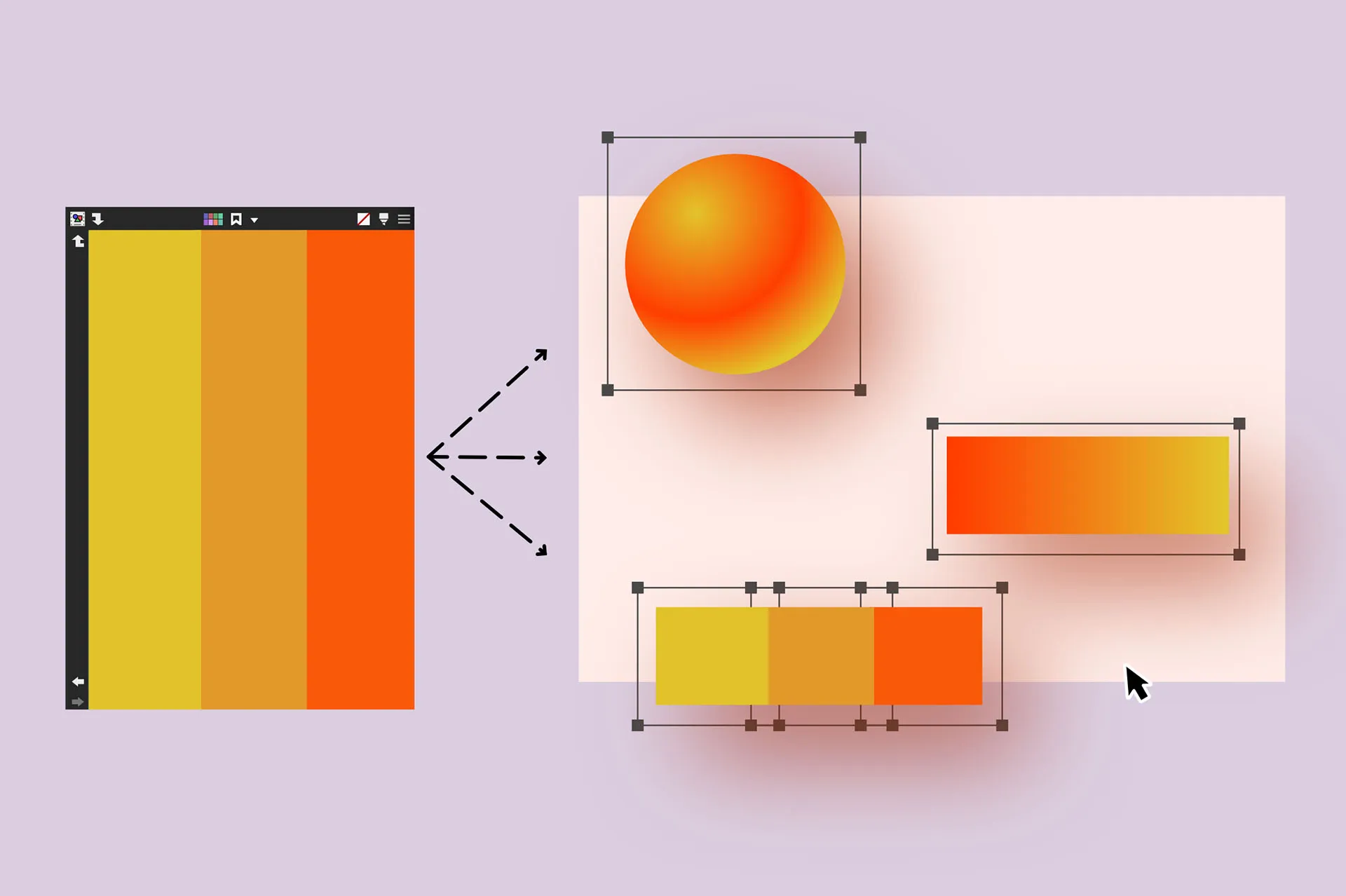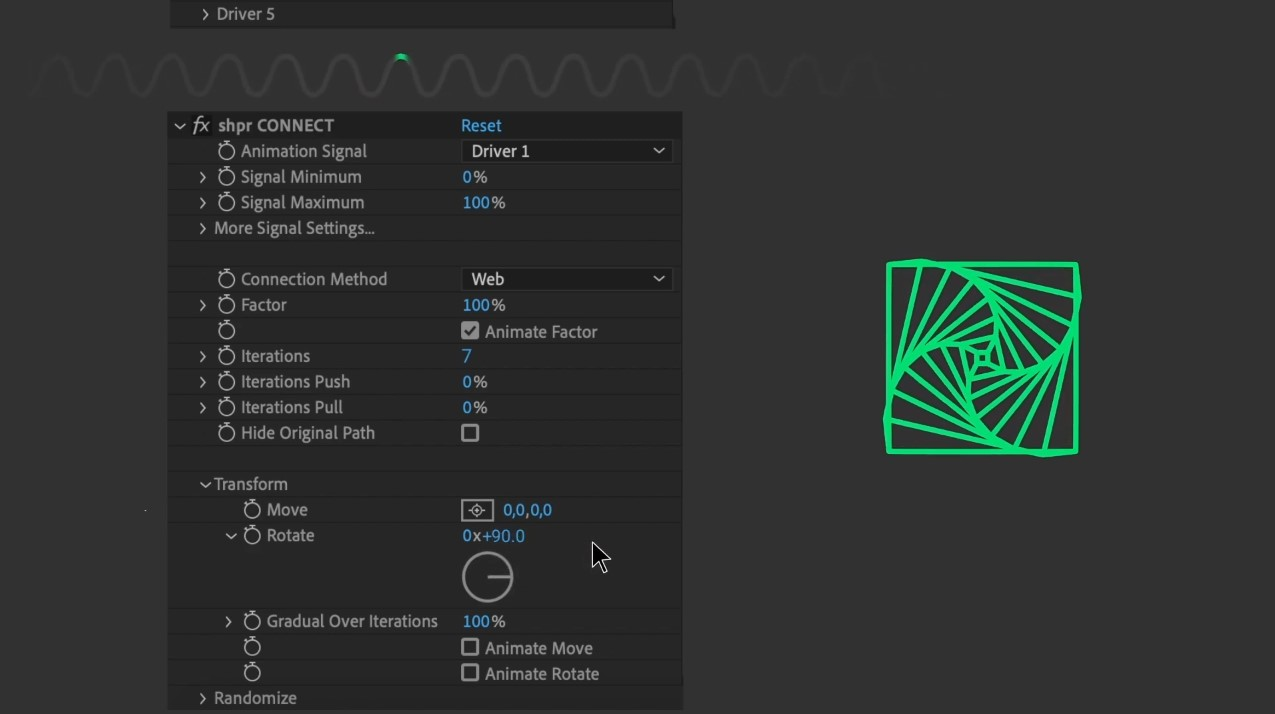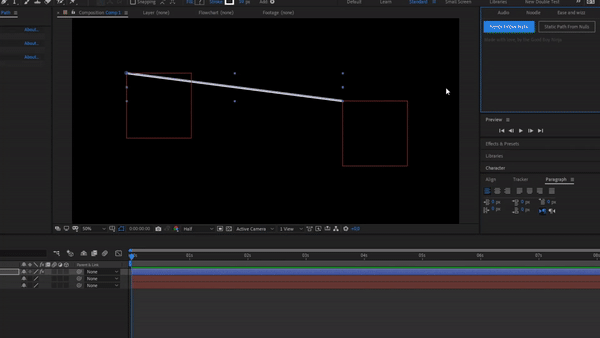# Copied!

May 25, 2020
After-Effects expression
Move along the edges of the comp

When applied to a position property, it places it on the edges of the comp. Use "time" as the percentage input like in the example below to drive the animation.

Good Boy Ninja
All Tools Sale
For a limited time
9
Days
99
Hours
99
Minutes
99
Seconds
Promo ends 30 Aug, 20:00 UTC
Null LayerPosition
Expression

function MoveAlongEdgesOfComp(percent, marginpercent) {

percent = (percent === undefined) ? 0 : percent % 100;

marginpercent = (marginpercent === undefined) ? 0 : clamp(marginpercent, 0, 100);

// convert the original percent value to both horizontal and vertical percent values to drive the motion

horizontalpercent = (percent >= 25 && percent <= 50) ? 100 : (percent >= 75 && percent <= 100) ? 0 : (percent < 25) ? (percent * 4) : (100 - (4 * (percent % 25)));

verticalpercent = (percent >= 0 && percent <= 25) ? 0 : (percent >= 50 && percent <= 75) ? 100 : (percent < 50) ? (percent - 25) * 4 : (100 - (4 * (percent % 25)));

midPoint = [thisComp.width * 0.5, thisComp.height * 0.5]; // use the linear function to drive each dimension

xLoc = linear(horizontalpercent, 0, 100, 0, thisComp.width);

yLoc = linear(verticalpercent, 0, 100, 0, thisComp.height);

finalPoint = [linear(marginpercent, 0, 100, xLoc, midPoint), linear(marginpercent, 0, 100, yLoc, midPoint), 0];

return finalPoint;

}

MoveAlongEdgesOfComp(time * 20, 50)

Also check out...WiggleColorsNoodleshprPaths from Nulls